Navigate back to the homepage

# Creating Water Trails with Vertex Displacement on a GLTF Model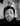Rick
September 19th, 2022 · 5 min read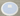So I initially wanted to investigate how to do vertex displacement and pass an array of mouse intersections with a plane to a custom shader. 💧💧💧

This then lead me to investigate onBeforeCompile and how to integrate my custom shader with a built in threejs material leveraging lighing and all the features of built in materials.

## The general flow is as follows:

• Create a basic model for testing

• Export hdri for use in r3f project

• Capture intersections with Plane

• Create a custom shader (very basic premise)

• Generate ripples algorithm at points of intersection
• Sum these ripples so it looks more realistic
• Decrease ripples with time and distance
• Integrate with a built in material - onBeforeCompile

• Update built in uniforms with useFrame

```.css-1chxjt6{position:absolute;right:22px;top:24px;padding:8px 12px 7px;border-radius:5px;color:#6f7177;-webkit-transition:background 0.3s ease;transition:background 0.3s ease;}.css-1chxjt6:hover{background:rgba(255,255,255,0.07);}.css-1chxjt6[data-a11y="true"]:focus::after{content:"";position:absolute;left:-2%;top:-2%;width:104%;height:104%;border:2px solid var(--theme-ui-colors-accent,#6166DC);border-radius:5px;background:rgba(255,255,255,0.01);}@media (max-width:45.9375em){.css-1chxjt6{display:none;}}1// Inspiration and useful guides:2//https://forum.unity.com/threads/re-map-a-number-from-one-range-to-another.119437/3//https://gist.github.com/yiwenl/3f804e80d0930e34a0b33359259b556c4// https://tympanus.net/codrops/2019/10/08/creating-a-water-like-distortion-effect-with-three-js/5// https://john-wigg.dev/DynamicWaterDemo/6// https://www.shadertoy.com/view/4dVcWR7//https://spectrum.chat/react-three-fiber/general/onbeforecompile-and-updating-uniforms~fd2d6306-11bd-4e9d-b323-e17f89ea99ec8import React, { useRef, useEffect, useCallback, useMemo } from "react";9import { useGLTF } from "@react-three/drei";10import { useFrame } from "@react-three/fiber";11import * as THREE from "three";12import "./styles.css";13
14let position = Array(100)15  .fill(1)16  .map((item) => ({17    origin: new THREE.Vector3(0, 0, 0),18    radius: 1019  }));20let formattedPosition = position.map(({ origin }) => origin);21let radi = position.map(({ radius }) => radius);22
23const customShader = {24  uniforms: {25    time: {26      value: 027    },28    positions: {29      value: formattedPosition30    },31    len: {32      value: 133    },34    radi: {35      value: radi36    },37    resolution: {38      value: new THREE.Vector2()39    }40  },41  vertexShader: {42    top: `43        #define STANDARD44        uniform float time;45        uniform vec3 positions;46        uniform int radi;47
48        float Remap (float value, float from1, float to1, float from2, float to2) {49            return (value - from1) / (to1 - from1) * (to2 - from2) + from2;50        }51  `,52
53    bottom: `54        float sum = 0.0;55        float velocity = 10.0;56        float total = 200.0;57
58        // We loop over the mouse intersections creating the 59        // cumulative displacement to make look more natural.60
61        for (int i = 0; i< 100;i++) {62            // Due to glsl not really allowing dynamic arrays63            // we pass a fixed length array with starting intersections64            // of 100 vec3(0.0, 0.0, 0.0).65
66            if (positions[i].x != 0.0) {67
68                // Calculate the diameter of the ripple using the decreasing radius 69                // Mapping to 0.0 - 0.8. This means the larger the radius the smaller 70                // the ripple, which is inline with a real water ripple.. ripples get larger.71
72                float diameter = 1.0 + Remap(float(radi[i]),total, 0.0, 0.0, 0.8);73
74                // The larger the index i gets the larger the value75                // which means the larger the diameter. So the older the 76                // mouse intersection with the plane the larger the diameter 77                // will be.78
79                float newRange = Remap(float(i), 0.0,100.0, 0.0, .80);80
81                // Comparison is what controls the diameter of the ripple, which increases82                // over time.83
84                float radiatingWidth = pow((diameter + newRange ), 2.0);85                86                // This is used to decrease the ripple back to zero87                float decreaseRipple = 1.0 + Remap(float(radi[i]),total, 0.0, .80, -.70);88                89                // Wave strength 90                float wave = 10.0;91                92                // Array of mouse positions (100) that intersect the plane93                vec3 mouse = positions[i].xyz;94                95                // Caculate the distance for each intersection from the current96                // vertex position to the mouse intersection. Allowing us to create a sin97                // wave from a specific point.98
99                float distanceToRipple =distance(position, mouse);100
101                // What size is the ripple? Is the distance to the ripple102                // within the bounds of the width of the ripple, if so we103                // calculate the displacement of the y coordinate. 104                if (distanceToRipple < radiatingWidth) {105                    // Calculate the actual ripple or displacement 106                    float ripple = cos(sin(distanceToRipple * wave - time * velocity));107
108                    // we want to decrease the strength of the wave over time and therefore109                    // the ripple strength 110                    wave -= decreaseRipple;111
112                    // Sum the ripples, all 100 of them. And decrease over time113                    // to diseminate the ripple 114                    sum += ripple * decreaseRipple;115
116                    // When we get to 0 by decreasing the sum of ripples and117                    // the strength of a ripple, we set to 0. So we add nothing118                    // to the original position119                    if (sum < 0.0) {120                        sum =0.0;121                    }122                    if (wave < 0.0) {123                        wave = 0.0;124                    }125                }126            }127        }128
129        // Add the overall displacement sum (tuning with a division)130        // to the Y of the current vertex position131        float y = position.y + sum/200.0;132
133        // And finally the usual matrix multiplications134        vec4 modelView = modelViewMatrix * vec4( position.x, y, position.z, 1.0);135        gl_Position = projectionMatrix *modelView;136    `137  }138};139
140// START OF COMPONENT141export const WaterPlane = () => {142  const increment = 1.0;143  const { nodes, materials } = useGLTF("/water-ripples-1.7.glb");144  const ref = useRef();145
146  useEffect(() => {147    setInterval(calc, 10);148  }, []);149
165  function calc() {166    const updated = position.map(({ origin, radius }, index) => {167      if (radius === 10) {168        return { origin, radius: 0 };169      }170      return { origin, radius: radius - increment };171    });172
173    position = updated.slice(0, 100);174    formattedPosition = position.map(({ origin }) => origin);175    radi = position.map(({ radius }, index) => radius);176  }177
178  const material = useMemo(() => {179    const m = new THREE.MeshStandardMaterial();180    m.onBeforeCompile = OBC; // Modifies the shader181    return m;182  }, [OBC]);183
190  return (191    <>192      <group dispose={null} ref={ref}>193        <mesh194          geometry={nodes.Sphere.geometry}195          material={nodes.Sphere.material}196        />197        <mesh geometry={nodes.Cube.geometry} material={nodes.Cube.material} />198        <mesh199          rotation={[0, 0, 0]}200          geometry={nodes.Plane002.geometry}201          material={materials["Material.001"]}202          onPointerMove={(e) => {203            if (position.length === 100) {204              position.unshift({205                origin: e.intersections.point,206                radius: 200.0207              });208            }209          }}210        >211          <primitive212            attach="material"213            object={material}214            {...materials["Material.001"]}215          />216        </mesh>217      </group>218    </>219  );220};```

## Create a basic model for testing

The model I made is very similar to others i make for testing, it involves a UV sphere. Scaling it first and then in edit mode we click the +z axis on the gizmo and look top down. Then select the top vertices, scale and translate.

This provides abit of a cup for the plane to sit, which will represent the water.

Here is the basic model I created: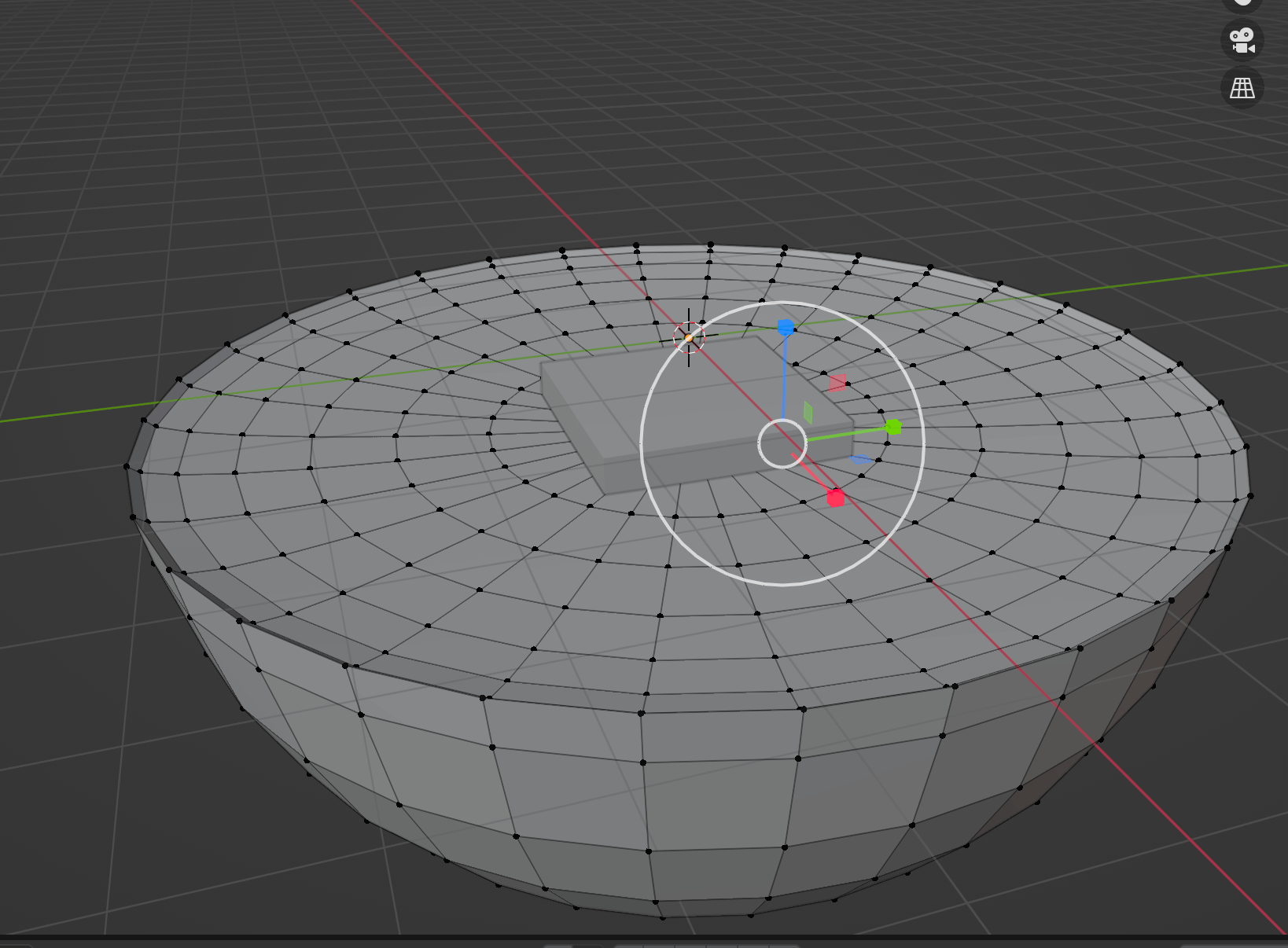I also whacked a cube in there for a point of reference.

Next we create a plane subdivide it until we get quite alot of verticies on the plane.

Move the plane down so it covers and intersects the outside of the custom UV sphere we made.

Use a boolean modifier with difference selected. We then apply the modifier.

Spilt the plane by loose parts, delete the outer loose bit and keep the middle bit which should now look like a circle type shape.

⚠️ N.B. Two points on vertices

1. If we dont have enough subdivides the vertices wont be uniform and evenly spreadout and when we displace them in the shader the movement will look clucky
2. If we dont have enough verticies no matter how strong the displacement, it will look like a very flat wave or ripple

⚠️ Before we export we need to crt + a and apply all transformations

We can and will export this to gltf and use gltfjsx with this command to get jsx output:

`1npx gltfjsx <modelName> -p 10`

The output should look something like this:

```1/*2Auto-generated by: https://github.com/pmndrs/gltfjsx3*/4
5import React, { useRef } from 'react'6import { useGLTF } from '@react-three/drei'7
8export function Model(props) {9  const { nodes, materials } = useGLTF('/water-ripples-1.5.glb')10  return (11    <group {...props} dispose={null}>12      <mesh geometry={nodes.Sphere.geometry} material={nodes.Sphere.material} />13      <mesh geometry={nodes.Cube.geometry} material={nodes.Cube.material} />14      <mesh geometry={nodes.Plane002.geometry} material={materials['Material.001']} />15    </group>16  )17}18

Right, now we have the model I simply gave the uv sphere base a light colour in the shading tab and followed this article to get some semi decent looking water / normal map.

Now we can move to the next step!

## Export hdri for use in r3f project

So I have a really cool addon for hdri’s, hdri maker blender addon. The really cool thing is you can either use some pretty good presets or create a shader texture for world background and then render a panoramic shot of the shaded texture and export as a hdri!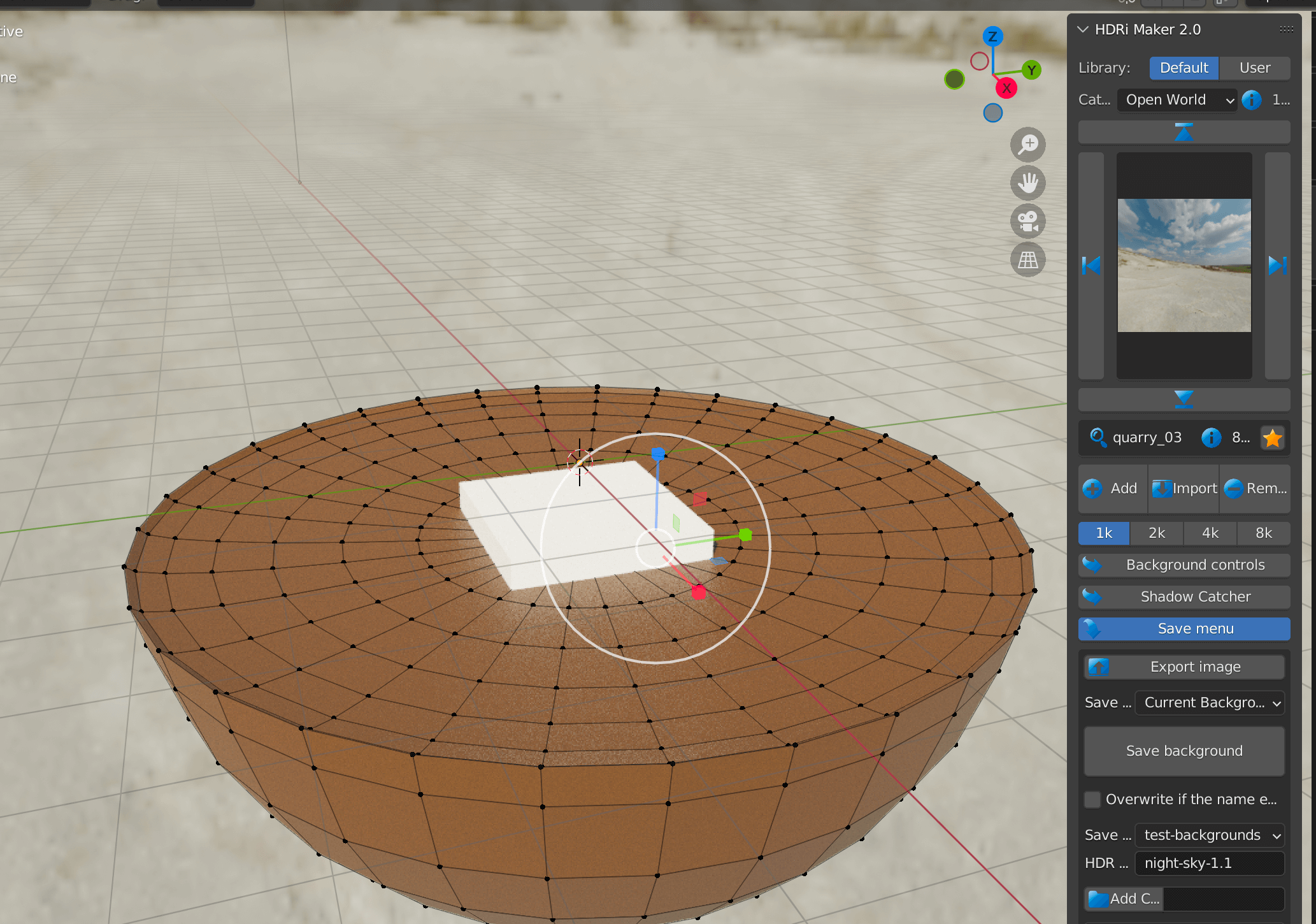You can export the present or save/render/export your own background as a hdri.

⚠️ Baring in mind hdri’s are light emitting, so the darker the scene the darker the objects in the scene. Dont forget to tweak the tone mapping and toneMapping exposure if you want to modify the intensity. Heres how you can do that:

`1<Canvas2    camera={{ position: [0, 5, 5] }}3    onCreated={({ gl }) => {4        gl.toneMappingExposure = 1.6;5        // gl.physicallyCorrectLights = true;6        gl.outputEncoding = "CineonToneMapping";7    }}8>`

You can then use Drei’s environment to load up the hdr.

`1<Environment2    background={true}3    files={"water-ripples-1.3.hdr"}4    path={"/"}5/>`

The background prop just tells r3f if you want the hdr as a background aswell. File name goes in files and then the path is just ’/’ which will be in the public folder.

So with our model and hdri done we can now focus on the shader and interaction.

## Capture intersections with Plane

First of if we want some kind of interaction with the plane then we are going to have to capture this.

Luckly with R3F, we have access to certain event listeners which accept a callback to run when the event is fired. Below shows what i have done:

`1<mesh2    rotation={[0, 0, 0]}3    geometry={nodes.Plane002.geometry}4    material={materials["Material.001"]}5    onPointerMove={(e) => {6    if (position.length === 100) {7        position.unshift({8        origin: e.intersections.point,9        radius: 200.010        });11    }12    }}13>14    <primitive15    attach="material"16    object={material}17    {...materials["Material.001"]}18    />19</mesh>`

This isnt mobile optimised but I use `onPointerMove` to add a intersection to the start of the positions array.

⚠️⚠️ A very important factor in why I setup an array initially with 100 origins and radi is because I couldnt get a workaround for dynamic arrays to work in glsl. As you have to define the length of the array. I know you can use `#define` and do the string repacement like so:

```1const shader = `2#define int length 1003
4uniform vec3 positions[length]5`6 // change the define 7
8useEffect(() => {9    shader.replace('100', dynamicLength)10}, [dynamicLength])```

But I just couldnt get this to work, so like the pragmatist I am, I decided plan B and create a initialized bus, older events === greater index in the array:

`1// Not using react state as we dont need to if all where we are2// using these variables is in useFrame.. + react couldnt handle 3// these fast updates4let position = Array(100)5  .fill(1)6  .map((item) => ({7    origin: new THREE.Vector3(0, 0, 0),8    radius: 109  }));10let formattedPosition = position.map(({ origin }) => origin);11let radi = position.map(({ radius }) => radius);`

The origin is where we will emenate the ripple from and the radius is like a modulator so the older added ripples will have a different radius to the ones just added. This is super important as we need something to say whats newer and whats older:

• older ripples = bigger

So we caputure the ripples or intersections via `onPointerMove` and we initialize the starting data. But how do we update this data, well heres my setup:

```1const increment = 10;2// setting interval every 10ms3useEffect(() => {4    setInterval(calc, 10);5}, []);  6
7// Calculation function 8function calc() {9    // Map over the position / intersection array to update10    const updated = position.map(({ origin, radius }, index) => {11        // and set radius to equal zero if the current radius is zero12        // so we dont get a negative radius13        if (radius === 0) {14        return { origin, radius: 0 };15        }16
17        // Otherwise we decrease the radius and return it.18        return { origin, radius: radius - increment };19    });20
21    // We only want a 100 items in the array as glsl cant handle dynamic arrays22    // or I couldn't figure it out23    position = updated.slice(0, 100);24
25    // Generate formatted data for passing to uniforms26    formattedPosition = position.map(({ origin }) => origin);27    radi = position.map(({ radius }, index) => radius);28}```

So we have a way of passing data to the shader but how do we construct the algorithm to actually do the ripples.

This was the hardest bit for me as Im still getting used to glsl 2 years on 😅

So we need to:

• Consume the intersections of the event handler in a uniform
• Loop over these positions
• Filter out the intial base vector data
• Create some modulators to add to the displacement of the current position y coordinate
• Is the distance from the current position/vertex less than the radiating width of the ripple? If so we want to allow us to displace and calculate the ripples displacement
• Cumulate or add up the total effect on the current position’s y coordinate
• Do the usual Matrix multiplications
```1float loop = 28.0;2    float sum = 0.0;3    float velocity = 10.0;4    float raio = 1.0;5    float total = 200.0;6
7    // We loop over the mouse intersections creating the 8    // cumulative displacement to make look more natural.9
10    for (int i = 0; i< 100;i++) {11
12      // Due to glsl not really allowing dynamic arrays13      // we pass a fixed length array with starting intersections14      // of 100 vec3(0.0, 0.0, 0.0).15
16      if (positions[i].x != 0.0) {17
18        // Calculate the diameter of the ripple using the decreasing radius 19        // Mapping to 0.0 - 0.8. This means the larger the radius the smaller 20        // the ripple, which is inline with a real water ripple.. ripples get larger.21
22        float diameter = 1.0 + Remap(float(radi[i]),total, 0.0, 0.0, 0.8);23
24        // The larger the index i gets the larger the value25        // which means the larger the diameter. So the older the 26        // mouse intersection with the plane the larger the diameter 27        // will be.28
29        float newRange = Remap(float(i), 0.0,100.0, 0.0, .80);30
31        // Comparison is what controls the diameter of the ripple, which increases32        // over time.33
34        float radiatingWidth = pow((diameter + newRange ), 2.0);35        36        // This is used to decrease the ripple back to zero37        float decreaseRipple = 1.0 + Remap(float(radi[i]),total, 0.0, .80, -.70);38        39        // Wave strength 40        float wave = 10.0;41        42        // Array of mouse positions (100) that intersect the plane43        vec3 mouse = positions[i].xyz;44        45        // Caculate the distance for each intersection from the current46        // vertex position to the mouse intersection. Allowing us to create a sin47        // wave from a specific point.48
49        float distanceToRipple =distance(position, mouse);50
51        // What size is the ripple? Is the distance to the ripple52        // within the bounds of the width of the ripple, if so we53        // calculate the displacement of the y coordinate.54
55        if (distanceToRipple < radiatingWidth) {56
57          // Calculate the actual ripple or displacement 58          float ripple = cos(sin(distanceToRipple * wave - time * velocity));59
60            // we want to decrease the strength of the wave over time and therefore61            // the ripple strength 62            wave -= decreaseRipple;63
64            // Sum the ripples, all 100 of them. And decrease over time65            // to diseminate the ripple 66            sum += ripple * decreaseRipple;67
68            // When we get to 0 by decreasing the sum of ripples and69            // the strength of a ripple, we set to 0. So we add nothing70            // to the original position71            if (sum < 0.0) {72              sum =0.0;73            }74            if (wave < 0.0) {75              wave = 0.0;76            }77        }78      }79    }80
81    // Add the overall displacement sum (tuning with a division)82    // to the Y of the current vertex position83    float y = position.y + sum/200.0;84
85    // And finally the usual matrix multiplications86    vec4 modelView = modelViewMatrix * vec4( position.x, y, position.z, 1.0);87    gl_Position = projectionMatrix *modelView;```

## Integrate with a built in material - onBeforeCompile

So we can make as many shaders as possible but the people who created the built in shaders / materials have decades of knowledge and it is unlikely you can do better than them! 👀

This means we need to find away to integrate our custom shader code into a built in material.

Welcome `.onBeforeCompile` which is documented here. It says: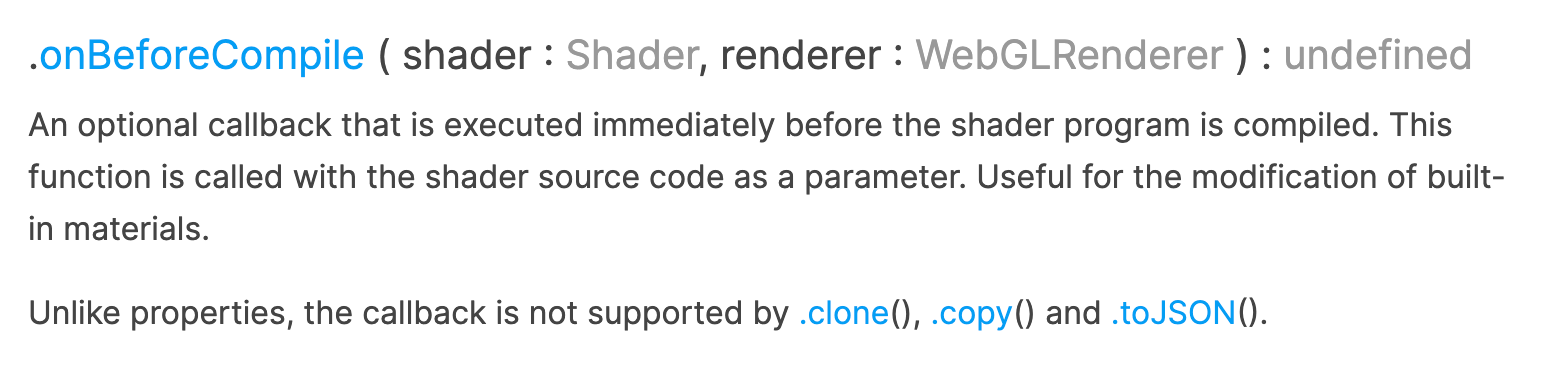This is brilliant, it says:
`Useful for the modification of built-in materials.`!

So we can inject this code into a built in material 😍

So here is my setup:

```1const OBC = useCallback((shader) => {2    console.log({ shader });3    // setShaderRef(shader);4    shader.uniforms = { ...shader.uniforms, ...customShader.uniforms };5
16const material = useMemo(() => {17    const m = new THREE.MeshStandardMaterial();18    m.onBeforeCompile = OBC; // Modifies the shader19    return m;20}, [OBC]);21
22// jsx 23
24<mesh25    rotation={[0, 0, 0]}26    geometry={nodes.Plane002.geometry}27    material={materials["Material.001"]}28    onPointerMove={(e) => {29    if (position.length === 100) {30        position.unshift({31            origin: e.intersections.point,32            radius: 200.033        });34    }35    }}36>37    <primitive38        attach="material"39        object={material}40        {...materials["Material.001"]}41    />42</mesh>```

This probably isnt an amazing setup but its one of the only ways I could get this to work.

First off We create an onBeforeCompile callback which will be called when we compile the shader.

And here we do string replacements with the builtin text and my custom shader.

How do you know what is in the orginal built in shader?

Well you can either search for the source code on sourceGraph or google the shaders behind the meshStandardMaterial.

Or a simpler way is to

`1console.log(shader)`

in onBeforeCompile and find a bit of string to replace.

Tbh I was probably alittle lazy as I just replaced the fog include, but you would probably want to replace something and then include the original string back in your shader.

And because I wanted my vertex code to be the last bit and not modified by anything I just plonked it at the end of the shader.

## Update built in uniforms with useFrame

This bit I genuinely found the hardest, updating the uniforms every frame. I only figured it out using this spectrum chat.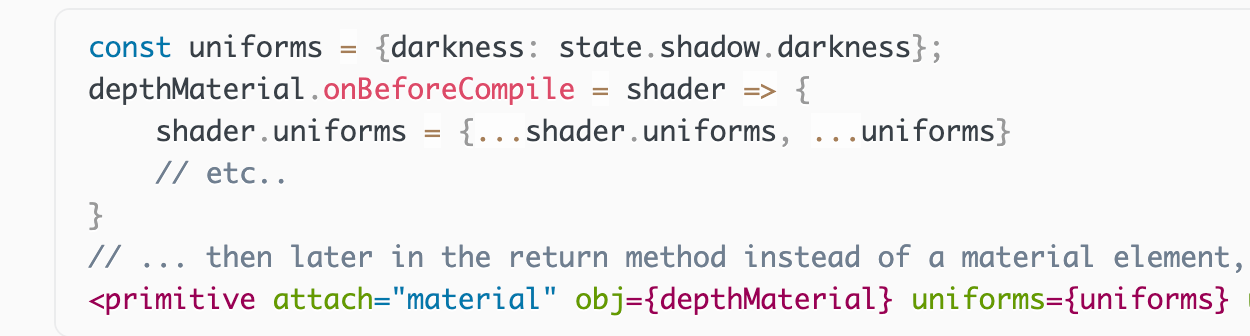I did it slightly different but I think the reason this works is we spread the new uniforms in `onBeforeCompile` and we then can basically update the original shader object uniforms which is referenced in the `onBeforeCompile` callback code.

Im not 100% sure on this so futher research would be required to give a definitive explanation.

## Final Thoughts

This is a cool little project which show cases several things:

• How to do water ripple tails
• How to integrate custom shader code into a built in material
• How to automatically use hdri/hdr images with custom shader code

The key thing here I think needs further work is to update the gl_FragColor in the fragment shader by calculating the normals in real time based off the displacement.

Cool effect though!

Until next time 🙂

### More articles from theFrontDev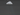### Creating light shafts from blender to a r3f project

A 101 on how to use geometry nodes and opacity masks in blender to generate some pretty cool looking light shafts for a threejs / react three fiber project.

September 4th, 2022 · 5 min read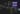### Storing positional x/y/z values in a texture and reading in a r3f shader material

This article will explore a workflow for storing vectors in an image and then reading it in a r3f shader material. Which can be used with three/r3f's points or GPU particles. Also this would be a good candiate for vector flow fields on the web.

September 2nd, 2022 · 5 min read